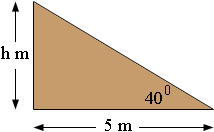SEARCH HOMEMath Central Quandaries & QueriesQuestion from carl: Width of my roof I am building is 5M at baseline, and the pitch is 40%. What will the height be, and how can I work this out in the future. Thanks for your help. Carl.Hi Carl,I let the height be h metres, then h/5 = tan(40o) and hence h = 5 × tan(40o) m. Make sure your calculator is set to degrees and use it to find tan(40o). Multiply by 5 to obtain the height in metres. I got 4.2 m.

PennyMath Central is supported by the University of Regina and The Pacific Institute for the Mathematical Sciences.